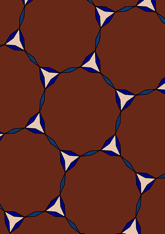# Derived from 3.12.12, with di-gons addeddata145/D312

## Geometry

• The symmetry group of the tiling is *632 (p6m).
• All the internal angles of the constituent polygons are a multiple of 7.5°.
• Contains two regular two-pointed star polygons with vertex angle of 22.5°.
• Contains one regular three-pointed star polygon with vertex angle of 37.5°.
• Contains one regular 12-pointed star polygon with vertex angle of 127.5°.
• The tiling satisfies the two-colour condition.
• The tiling is edge-to-edge.
• As drawn, contains about 142 polygons.

## References

Publications referenced:
1. Uniform Star Polygons with Digons of B A Wichmann. The World of Patterns, World Scientific, 2001. ISBN 981024619. [wich2] {Only new patterns ascribed to this.}

v53